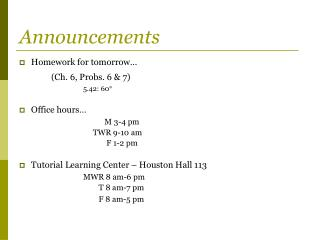# Announcements - PowerPoint PPT PresentationDownload PresentationAnnouncements

AnnouncementsDownload Presentation## Announcements

- - - - - - - - - - - - - - - - - - - - - - - - - - - E N D - - - - - - - - - - - - - - - - - - - - - - - - - - -
##### Presentation Transcript

1. Announcements • Homework for tomorrow… (Ch. 6, Probs. 6 & 7) 5.42: 60° • Office hours… M 3-4 pm TWR 9-10 am F 1-2 pm • Tutorial Learning Center – Houston Hall 113 MWR 8 am-6 pm T 8 am-7 pm F 8 am-5 pm

2. Chapter 6 Identifying and Using Forces (Normal Force and Tension)

3. Problem 5.57 A boy whirls a stone in a horizontal circle of radius 1.5 m and at a height 2.0 m above level ground. The string breaks, and the stone flies off horizontally and strikes the ground after traveling a horizontal distance of 10 m. What is the magnitude of the centripetal acceleration of the stone while in circular motion?

4. Newton’s 2nd Law in 3D Newton’ 2nd Law is a vector equation… Newton’s 2nd Law can be written in terms of it’s components

5. Some particular forces… • Gravitational force ( ) • Normal force ( ) • Tension force ( ) • Frictional force ( )

6. How to solve “F=ma” problems… • Pick a body • Draw free-body diagram • Choose a coordinate system • Break forces into components & use Newton’s 2nd

7. Quiz Question 1: Two forces are shown acting on an object. For the object not to accelerate, a 3rd force must be applied to the object that has a magnitude equal to: • 1N • 3N • 4N • 5N • 7N 3N 4N

8. Normal Force: Question An object of mass m rests on the table. What is the force of the table on the object? (What is the ‘normal force’, ? ) • is perpendicular to the surface

9. Quiz Question 2: The figure below shows 2 equal 100 lb. weights connected by a light string and supported by a pulley. The tension in the string is: • 0 lbs • 50 lbs • 100 lbs • 200 lbs • It is impossible to know from the data given m1

10. Tension Comment: • For a rope of negligible mass, the magnitude of the force exerted on a rope is the same at all points on the rope

11. Quiz Question 3 Consider the two situations shown in which there is no acceleration. In both cases the men pull with a force of magnitude F. Is the reading on the scale in part (i) of the figure • greater than • less than • equal to the reading in (ii)?

12. Example 1: What is the acceleration of mass down the inclined plane? What is the Normal force? (In this problem, friction is negligible)